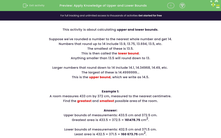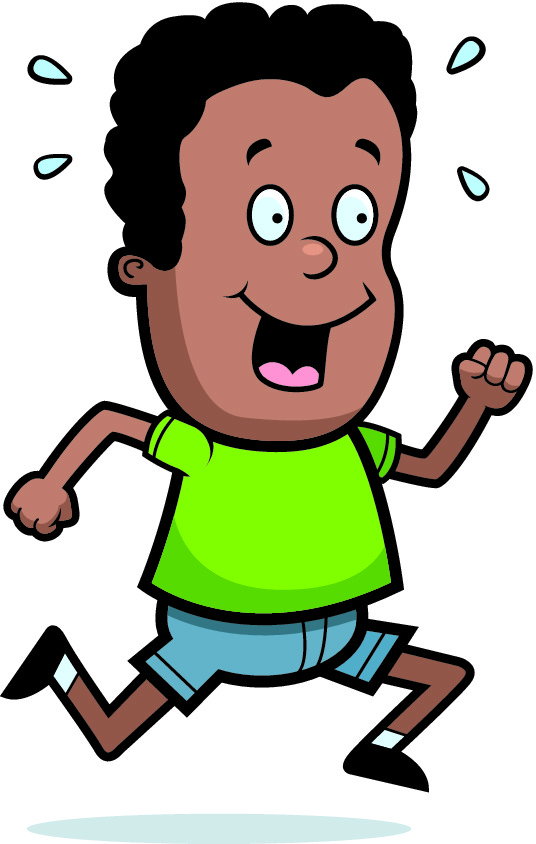# Apply Knowledge of Upper and Lower Bounds

In this worksheet, students will use upper and lower bounds in order to calculate areas and speeds from the given information.Key stage:  KS 3

Curriculum topic:   Number

Curriculum subtopic:   Round/Approximate Numbers Appropriately

Popular topics:   Estimation worksheets

Difficulty level:#### Worksheet Overview

This activity is about calculating upper and lower bounds.

Suppose we've rounded a number to the nearest whole number and got 14.

Numbers that round up to 14 include 13.9, 13.75, 13.694, 13.5, etc.

The smallest of these is 13.5.

This is then called the lower bound.

Anything smaller than 13.5 will round down to 13.

Larger numbers that round down to 14 include 14.1, 14.34968, 14.49, etc.

The largest of these is 14.4999999...

This is the upper bound, which we write as 14.5.

Example 1:

A room measures 433 cm by 372 cm, measured to the nearest cm.

Find the greatest and smallest possible areas of the room.

Upper bounds of measurements: 433.5 cm and 372.5 cm.

Greatest area is 433.5 × 372.5 = 161,478.75 cm2.

Lower bounds of measurements: 432.5 cm and 371.5 cm.

Smallest area is 432.5 × 371.5 = 160,673.75 cm2.

Example 2:

A sprinter runs 100 m in 12.8 seconds.

The distance is measured to the nearest cm and the time to the nearest tenth of a second.

Find the fastest and slowest possible speeds of the sprinter.

Upper bounds of measurements: 100.005 m and 12.85 s

Lower bounds of measurements: 99.995 m and 12.75 s.

The formula for finding speed is: speed = distance ÷ time

Be careful here!

For the fastest speed, we want the maximum distance in the minimum time:

The fastest speed = 100.005 ÷ 12.75 = 7.84 m/s (3 s.f.)

For the slowest speed, we want the minimum distance in the maximum time:

The slowest speed = 99.995 ÷ 12.85 = 7.78 m/s (3 s.f.)Now it's over to you.

### What is EdPlace?

We're your National Curriculum aligned online education content provider helping each child succeed in English, maths and science from year 1 to GCSE. With an EdPlace account you’ll be able to track and measure progress, helping each child achieve their best. We build confidence and attainment by personalising each child’s learning at a level that suits them.

Get started